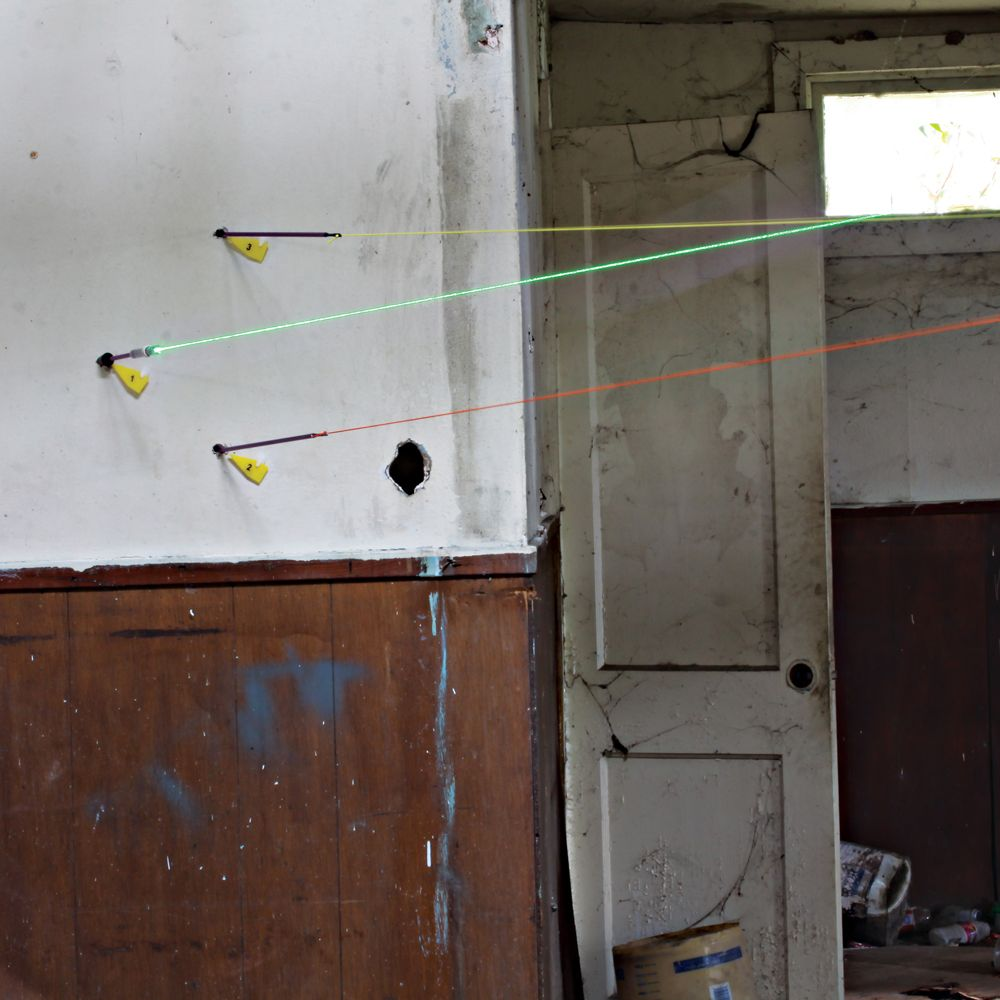# Ballistics Investigation ActivityBallistics is the study of projectile motion and a critical area of investigation in forensic study. When a projectile is fired from a rifle or handgun, it travels at a specific velocity. A projectile in motion can be described by both horizontal and vertical motions. The motion in two directions is represented mathematically as a parabola.

For projectiles that travel less than 20 meters, the effect of gravity is negligible, so forensic scientists use a straight line to represent the projectile path. The analysis then uses right triangle trigonometry instead of the entire parabola. Forensic scientists know the atmosphere (including wind and humidity) may affect the trajectory of a projectile.

### How are the trajectory calculations completed?

If a projectile enters an object at a 90-degree angle to the surface, the projectile will make a circular hole. If the projectile penetrates at an angle, it will leave an elliptical hole. Based on the geometry of the ellipse, we can determine the angle of entry of the projectile. To determine angle of entry, the investigator measures the minor axis of the ellipse and the major axis of the ellipse. The major axis of the ellipse becomes the hypotenuse of a right triangle, and the width becomes the opposite side of a right triangle. Using the sine function, the angle of incidence of the bullet can be calculated.

Angle of incidence is calculated as: sin Ï´ = minor axis/major axis

### Essential question

How can holes left by projectiles aid forensic investigations?

### Investigation objectives

Calculate the angle of incidence given a projectile hole.

### Safety

Use rulers properly.

### Materials

Each student should have:

• Ballistics Student Investigation Sheet
• Metric Ruler
• Calculator with Trigonometry Functions

### Activity

Below is a set of practice termination perforations. Measure the minor axis and major axis in centimeters and then calculate the angle of incidence.

Angle of incidence is calculated as: sin Ï´ = minor axis/major axis

Now that you know how to calculate the angle of incidence, you can extend your calculations to find the location of the shooter.